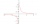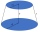# Two rectangles 2

A square of area 36 cm2 is cut out to make two rectangles. A and B The area of area A to area B is 2 : 1 Find the dimensions of rectangles A and B.

Result

a =  4 cm
b =  6 cm
c =  2 cm
d =  6 cm

#### Solution:

$S = 36 \ cm^2 \ \\ S = x^2 \ \\ \ \\ x = \sqrt{ S } = \sqrt{ 36 } = 6 \ cm \ \\ \ \\ S_{ 1 } = \dfrac{ 2 }{ 2+1 } \cdot \ S = \dfrac{ 2 }{ 2+1 } \cdot \ 36 = 24 \ cm^2 \ \\ S_{ 2 } = \dfrac{ 1 }{ 2+1 } \cdot \ S = \dfrac{ 1 }{ 2+1 } \cdot \ 36 = 12 \ cm^2 \ \\ \ \\ a = S_{ 1 }/x = 24/6 = 4 = 4 \ \text { cm }$
$b = x = 6 = 6 \ \text { cm }$
$c = S_{ 2 }/x = 12/6 = 2 = 2 \ \text { cm }$
$d = x = 6 = 6 \ \text { cm }$

Leave us a comment of this math problem and its solution (i.e. if it is still somewhat unclear...):Be the first to comment!## Next similar math problems:

1. A rectangle 2A rectangle has a diagonal length of 74cm. It’s sides are 5:3. Find its lengths.
2. Coordinates of square verticesI have coordinates of square vertices A / -3; 1/and B/1; 4 /. Find coordinates of vertices C and D, C 'and D'. Thanks Peter.
3. Secret treasureScouts have a tent in the shape of a regular quadrilateral pyramid with a side of the base 4 m and a height of 3 m. Determine the radius r (and height h) of the container so that they can hide the largest possible treasure.
4. Folding tableThe folding kitchen table has a rectangular shape with an area of 168dm2 (side and is 14 dm long). If necessary, it can be enlarged by sliding two semi-circular plates (at sides b). How much percent will the table area increase? The result round to one-hun
5. Heptagonal pyramidA hardwood for a column is in the form of a frustum of a regular heptagonal pyramid. The lower base edge is 18 cm and the upper base of 14 cm. The altitude is 30 cm. Determine the weight in kg if the density of the wood is 0.10 grams/cm3.
6. Surface of the cylinderCalculate the surface of the cylinder for which the shell area is Spl = 20 cm2 and the height v = 3.5 cm
7. Sides of right angled triangleOne leg is 1 m shorter than the hypotenuse, and the second leg is 2 m shorter than the hypotenuse. Find the lengths of all sides of the right-angled triangle.
8. A rhombusA rhombus has sides of length 10 cm, and the angle between two adjacent sides is 76 degrees. Find the length of the longer diagonal of the rhombus.
9. Equation 23Find value of unknown x in equation: x+3/x+1=5 (problem finding x)
10. Before yesterdayHe merchant adds a sale sign in his shop window to the showed pair of shoes in the morning: "Today by p% cheaper than yesterday. " After a while, however, he decided that the sign saying: "Today 62.5% cheaper than the day before yesterday". Determine the n
11. Compound interest 3After 8 years, what is the total amount of a compound interest investment of \$25,000 at 3% interest, compounded quarterly? (interest is now dream - in the year 2019)
12. Geography testsOn three 150-point geography tests, you earned grades of 88%, 94%, and 90%. The final test is worth 250 points. What percent do you need on the final to earn 93% of the total points on all tests?
13. Isosceles triangle 10In an isosceles triangle, the equal sides are 2/3 of the length of the base. Determine the measure of the base angles.
14. The hemisphereThe hemisphere container is filled with water. What is the radius of the container when 10 liters of water pour from it when tilted 30 degrees?
15. Frustum of a coneA reservoir contains 28.54 m3 of water when completely full. The diameter of the upper base is 3.5 m while at the lower base is 2.5 m. Determine the height if the reservoir is in the form of a frustum of a right circular cone.
16. Cylinder and its circumferenceIf the height of a cylinder is 4 times its circumference. What is the volume of the cylinder in terms of its circumference, c?
17. Medians in right triangleIt is given a right triangle, angle C is 90 degrees. I know it medians t1 = 8 cm and median t2 = 12 cm. .. How to calculate the length of the sides?